Web Programming TutorialsLearn Closure Call and Filtered Unserialize Methods in PHP 7

# Learn Closure Call and Filtered Unserialize Methods in PHP 7In the last chapter, we discussed about new PHP 7 feature “anonymous classes” with examples. In this chapter, we are going to discuss about new special methods known as ‘closure call’ and ‘filtered unserialize’ that are added to PHP 7.

Closure call () vs bindTo ()
PHP language has support to bind an object scope of a class to a closure and then invoke it. This can be achieved by using the ‘bindTo’ method in PHP 5.x as shown below.
\$value = \$getValue->bindTo (new Math, ‘Math’);

In PHP 7, above method is replaced by closure ‘call’ method. Following is the syntax for this new method.
\$value = \$getValue->call (new Math);

Example 1:
Let’s understand ‘bindTo’ methods with following example PHP code.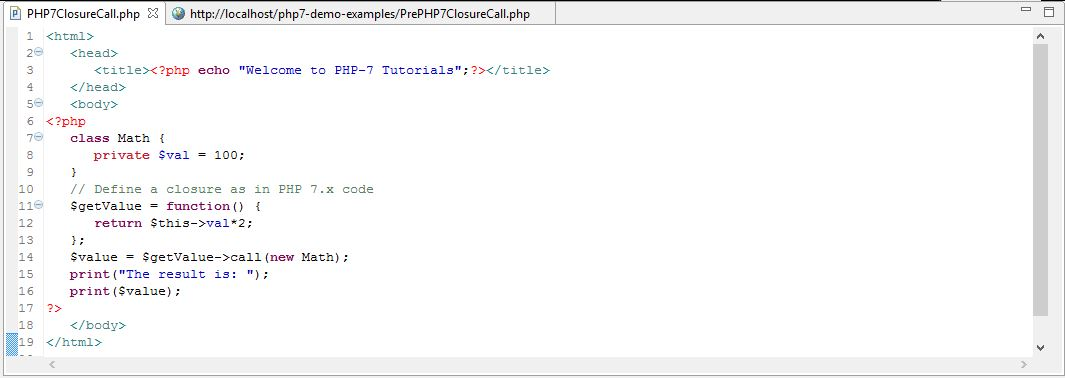Explanation of above PHP code

• Firstly, we are defining a class ‘Math’ that has one data member variable as ‘\$val’.
• Secondly, we are defining a closure method that return the multiply product of the variable ‘\$val’ and 2.
• Next, we are binding above closure method using ‘bindTo’ function and passing instance of class ‘Math’ and class name as ‘Math’ as arguments to this method.
• This is the way we do binding of closure method in PHP 5.x.
• Lastly, when we print the closure method as ‘\$value ()’, it will print the product of 100 (value of variable) and 2 i.e. 200.

Output
Following output displays the product value of 200 achieved through closure method binding using ‘bindTo’ method.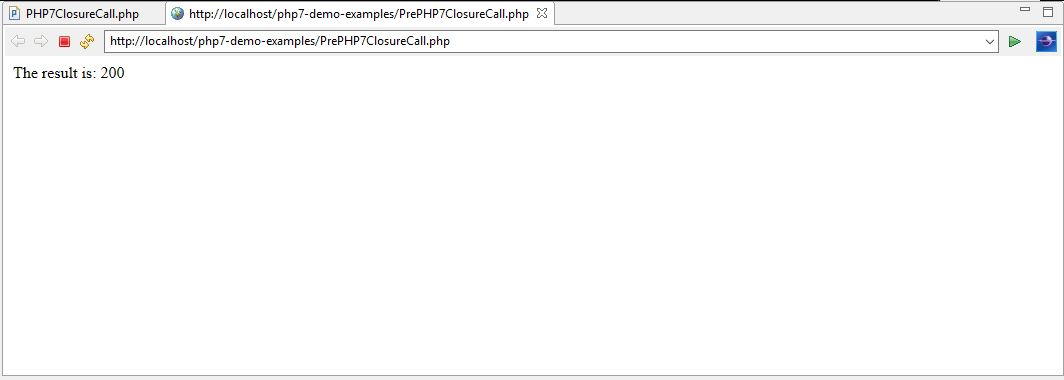Example 2:
Let’s understand ‘call’ methods with following example PHP 7 code.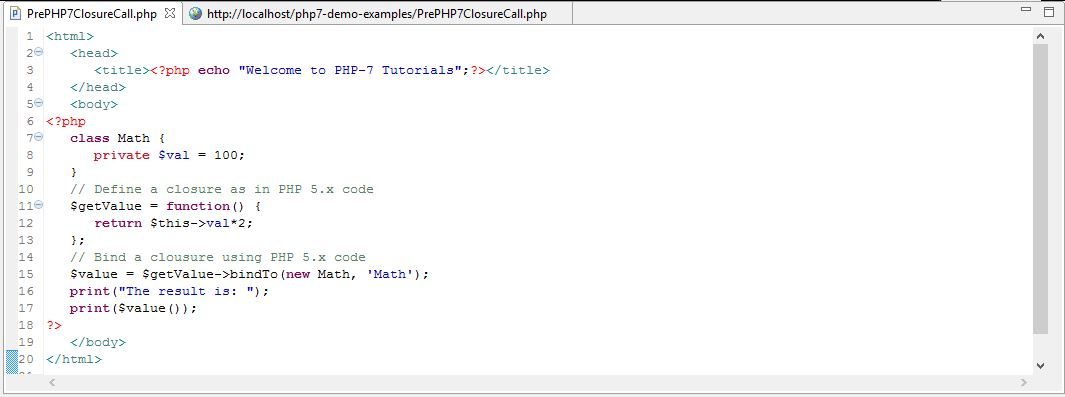Explanation of above PHP 7 code

• Firstly, we are defining a class ‘Math’ that has one data member variable as ‘\$val’.
• Secondly, we are defining a closure method that returns the multiplication product of the variable ‘\$val’ and 2.
• Next, we are binding the above closure method using ‘call’ function and passing instance of class ‘Math’ only as an argument to this method.
• This is the way we do binding of closure method in PHP 7.x.
• Lastly, when we print the closure method as ‘\$value’, it will print the product of 100 (value of variable) and 2 i.e. 200.

Output
Following output displays the product value of 200 as achieved through closure method binding using ‘call’ method that is added to PHP 7.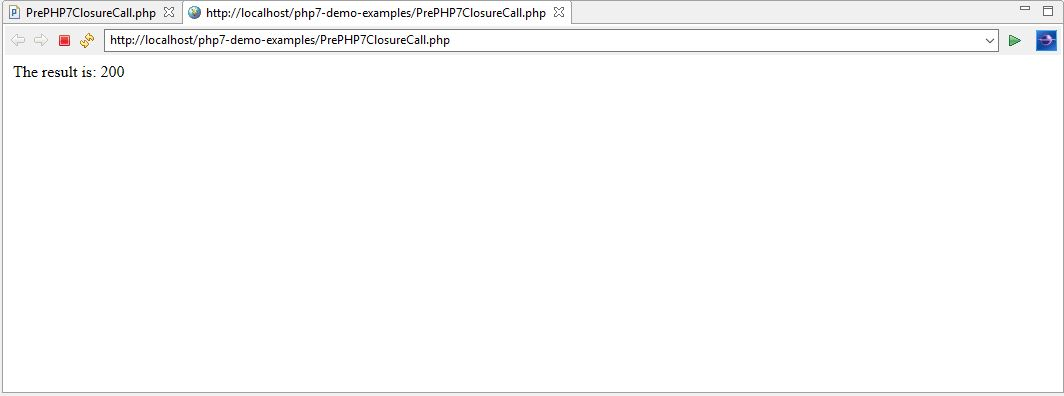Comparison between ‘bindTo’ and ‘call’ method

• The ‘bindTo’ method that was used in PHP 5.x versions whereas the ‘call’ closure method has recently added to PHP 7.
• The ‘bindTo’ method accepts two arguments. The first argument is the instance of a class and the second argument is the name of that class whereas the ‘call’ method accepts only one argument, the instance of a class.
• While printing the reference of a closure method using ‘bindTo’ method, we are printing as ‘print (\$value ())’. Notice the function parentheses which returns the string object. However, using ‘call’ method, we can print either as ‘print (\$value ())’ or ‘print (\$value)’e. with or without parentheses.

Filtered unserialize ()
PHP supports a method known as ‘serialize (Object)’ that is used to serialize the object. In PHP 7, an additional security feature has been added by introducing filtering ‘unserialize (arg1, arg2)’ method. It is used to filter out objects that are unserialized on untrusted data. This helps in preventing possible code injections that enables the PHP programmers to whitelist or blacklist classes based on serialization.

Syntax for filtered unserialize (arg1, [arg2])
\$data = unserialize (\$serializObject1, [“allowed_classes” => true]);
In the above syntax, the first argument is always the serialized object and is mandatory. If we do not pass a serialized object as the first argument then the PHP 7 parser will throw a runtime exception as ‘unserialize () expects parameter 1 to be string’. Second argument is optional and if passed, it allows adding additional filter criteria on the serialized class object that is passed as the first argument depending on the following values.

• If the second argument is passed as ‘[“allowed_classes” => true])’, unserialize method accepts all classes. It is the default behaviour where the argument 2 value is omitted.
• If the second argument is passed as ‘[“allowed_classes” => false])’, unserialize method will converts all objects into ‘__PHP_Incomplete_Class’
• If the second argument is passed as ‘[“allowed_classes” => [“MyPHP7Class1”, “MyPHP7Class2”]])’, unserialize method will allow the serialized object (in the first argument) to be among these classes otherwise it will convert all objects into ‘__PHP_Incomplete_Class’

Example 3:
Let’s understand ‘unserialize’ methods with following example PHP 7 code.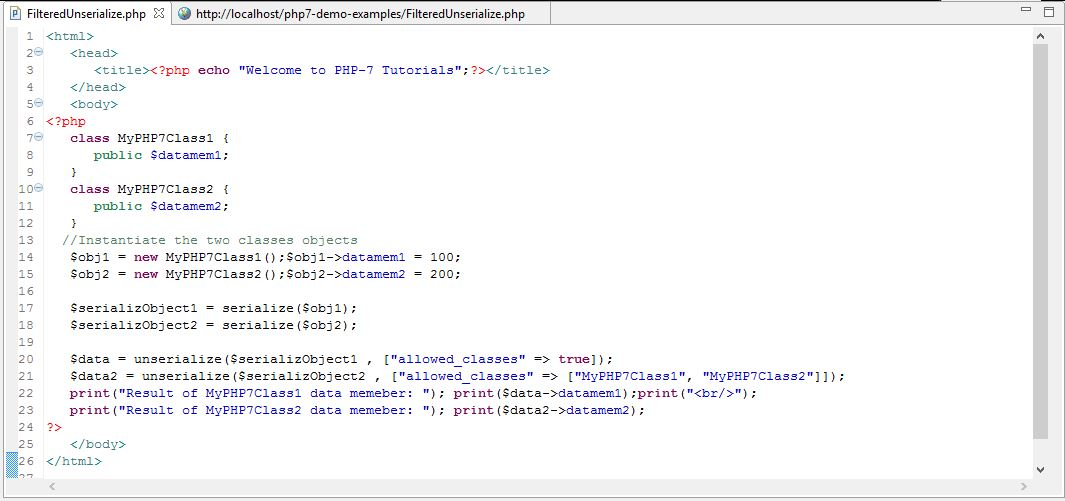Explanation of above PHP 7 code

• Firstly, we have defined two classes namely MyPHPClass1 and MyPHPClass1. Each of these class has one data member variable defined as ‘\$datamem1’ and ‘\$datamem2’
• Secondly, we are instantiating these two classes as objects namely ‘\$obj1’ and ‘\$obj2’. After creating these objects, we are calling and assigning data members ‘\$datamem1’ and ‘\$datamem2’ values as 100 and 200 respectively.
• Thirdly, we are serializing both objects ‘\$obj1’ and ‘\$obj2’ by using PHP ‘serialize’ method as shown above. This will result in serialized objects as ‘\$serializeObject1’ and ‘\$serializeObject2’
• In the first use of filtered ‘unserialize’ method, we are passing first argument as serialized object ‘\$serializeObject1’ and second argument as ‘[“allowed_classes” => true])’. The result of filter condition is very obvious as this method can accept all serialized classes. Therefore, the reference of this method is passed to new ‘\$data’ When we print the data member associated with object (\$data->datamem1), it will print the value as 100.
• In the second use of filtered ‘unserialize’ method, we are passing first argument as serialized object ‘\$serializeObject2’ and second argument as ‘[“allowed_classes” => [“MyPHP7Class1”, “MyPHP7Class2”]]’. The result of filter condition is very obvious as this method can accept both classes of which this object belongs to class ‘MyPHP7Class2’. Therefore, the reference of this method is passed to new ‘\$data2’ When we print the data member associated with object (\$data2->datamem2), it will print the value as 200.

Output
Following is the output of the above PHP 7 program that displays the output which we just discussed above.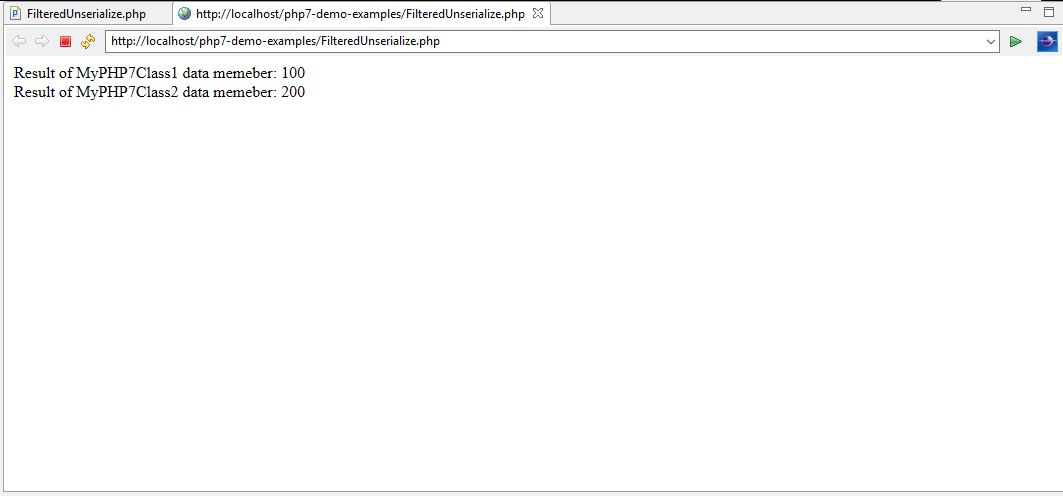Conclusion
In this chapter, we explored new special methods, ‘closure call’ and ‘filtered unserialize’ that are added to PHP 7 as oppose to PHP 5.x. In the next chapter, we are going to discuss about IntlChar class which is another new feature that has added to PHP 7.

### How Challenging Is B2B Marketing?

21,501Fans
4,218Followers
103,000Subscribers

### How Challenging Is B2B Marketing?

- Advertisement -